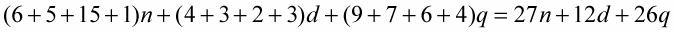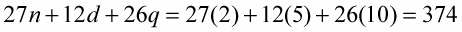##### Algebra I For DummiesAlgebra simplifies expressions with coefficients, which are numbers preceding variables. For example, 3 is the coefficient in 3x. Rather than using a multiplication sign between 3 and x, the number is just written in front of a variable, which means that the 3 and x are multiplied together. Instead of writing a + a + a + a, you can express it as 4a, with 4 being the coefficient.

When several variables are multiplied together, multiplication symbols aren’t needed. The term 3xyz means that all four factors are multiplied together.

You can use variables to simplify the following exercise: Collect, organize, and report on the coins collected during a charity drive.

Let n represent the value of the nickels in a roll of nickels.

Let d represent the value of the dimes in a roll of dimes.

Let q represent the value of the quarters in a roll of quarters.

2. After collecting the money, you get the following information from your helpers:

Ann collected six rolls of nickels, four rolls of dimes, and nine rolls of quarters, or 6n + 4d + 9q.

Ben collected five rolls of nickels, three rolls of dimes, and seven rolls of quarters, or 5n + 3d + 7q.

Cal collected 15 rolls of nickels, two rolls of dimes, and six rolls of quarters, or 15n + 2d + 6q.

Don collected one roll of nickels, three rolls of dimes, and four rolls of quarters, or n + 3d + 4q.

3. Set up an equation to add all the rolls of nickels, dimes, and quarters:4. Compute the total amount of money raised.

A roll of nickels is worth \$2, so n = 2.

A roll of dimes is worth \$5, so d = 5.

A roll of quarters is worth \$10, so q = 10.A total of \$374 was raised.